libsnark 是目前实现 zk-SNARKs 电路最重要的框架，在众多私密交易或隐私计算相关项目间广泛应用，其中最著名当然要数 Zcash。Zcash 在 Sapling 版本升级前一直使用 libsnark 来实现电路（之后才替换为 bellman）。毫不夸张地说，libsnark 支撑并促进了 zk-SNARKs 技术的首次大规模应用，填补了零知识证明技术从最新理论到工程实现间的空缺。

## 1. zk-SNARKs 和 libsnark 背景简介

zk-SNARKs 能应用到很多场景，比如隐私保护、区块链扩容、可验证计算等。本文不介绍 zk-SNARKS 和零知识证明的理论细节，不熟悉或想深入了解的同学可阅读其他文章或论文。

• [GGPR13] Quadratic span programs and succinct NIZKs without PCPs , Rosario Gennaro, Craig Gentry, Bryan Parno, Mariana Raykova, EUROCRYPT 2013

• [PGHR13] Pinocchio: Nearly Practical Verifiable Computation , Bryan Parno, Craig Gentry, Jon Howell, Mariana Raykova, IEEE Symposium on Security and Privacy (Oakland) 2013

• [BCGTV13] SNARKs for C: Verifying Program Executions Succinctly and in Zero Knowledge , Eli Ben-Sasson, Alessandro Chiesa, Daniel Genkin, Eran Tromer, Madars Virza, CRYPTO 2013

• [BCIOP13] Succinct non-interactive arguments via linear interactive Proofs , Nir Bitansky, Alessandro Chiesa, Yuval Ishai, Rafail Ostrovsky, Omer Paneth, Theory of Cryptography Conference 2013

• [BCTV14a] Succinct non-interactive zero knowledge for a von Neumann architecture , Eli Ben-Sasson, Alessandro Chiesa, Eran Tromer, Madars Virza, USENIX Security 2014

• [BCTV14b] Scalable succinct non-interactive arguments via cycles of elliptic curves , Eli Ben-Sasson, Alessandro Chiesa, Eran Tromer, Madars Virza, CRYPTO 2014

• [Groth16] On the Size of Pairing-based Non-interactive Arguments , Jens Groth, EUROCRYPT 2016

libsnark 的开发者们亦是这个领域内顶尖的学者或研究牛人，如 Eran Tromer 更是以上多篇论文的共同作者。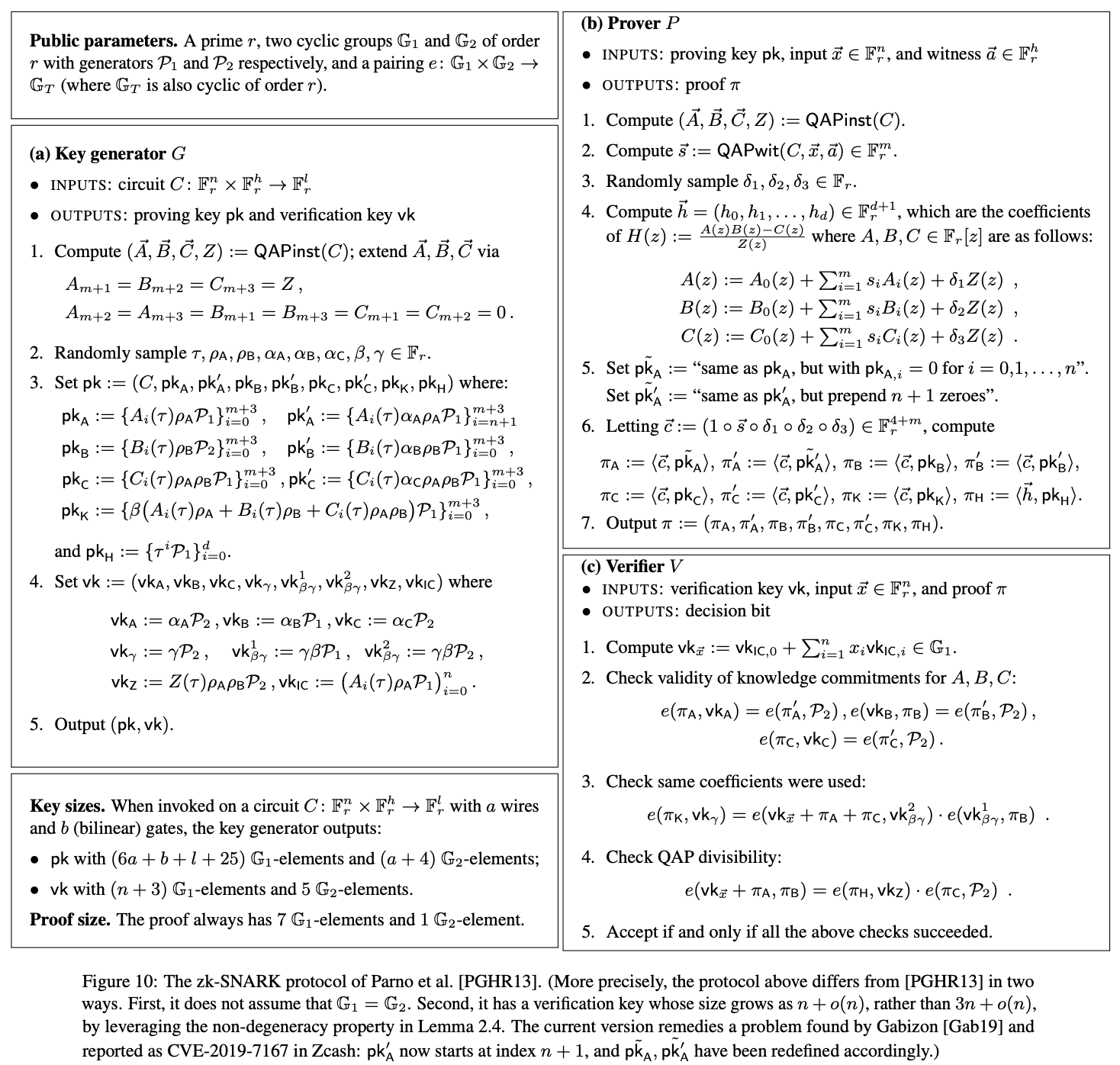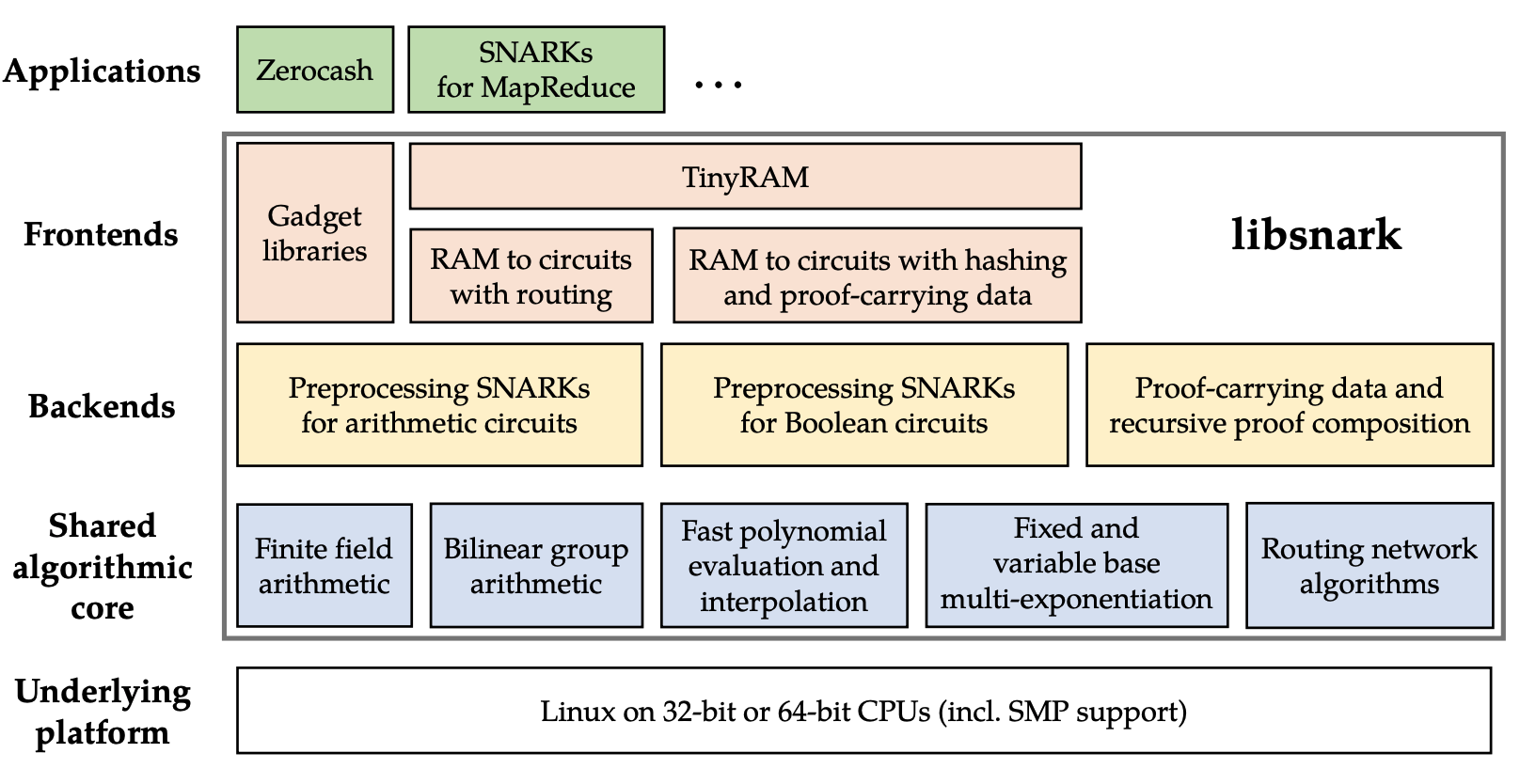)

libsnark 框架提供了多个通用证明系统的实现，其中使用较多的是 BCTV14a 和 Groth16。

• zk_proof_systems/ppzksnark/r1cs_ppzksnark 对应的是 BCTV14a
• zk_proof_systems/ppzksnark/r1cs_gg_ppzksnark 对应的是 Groth16

## 2. 基本原理与步骤

1. 将待证明的命题表达为 R1CS (Rank One Constraint System)
2. 使用生成算法（G）为该命题生成公共参数
3. 使用证明算法（P）生成 R1CS 可满足性的证明
4. 使用验证算法（V）来验证证明

 1 2 3  function C(x, out) { return ( x^3 + x + 5 == out ); } 

 1 2 3  lambda <- random() (pk, vk) = G(C, lambda) 

 1  proof = P(pk, out, x) 

 1  V(vk, out, proof) ?= true## 3. 搭建 zk-SNARKs 应用开发环境

 1  git clone https://github.com/sec-bit/libsnark_abc.git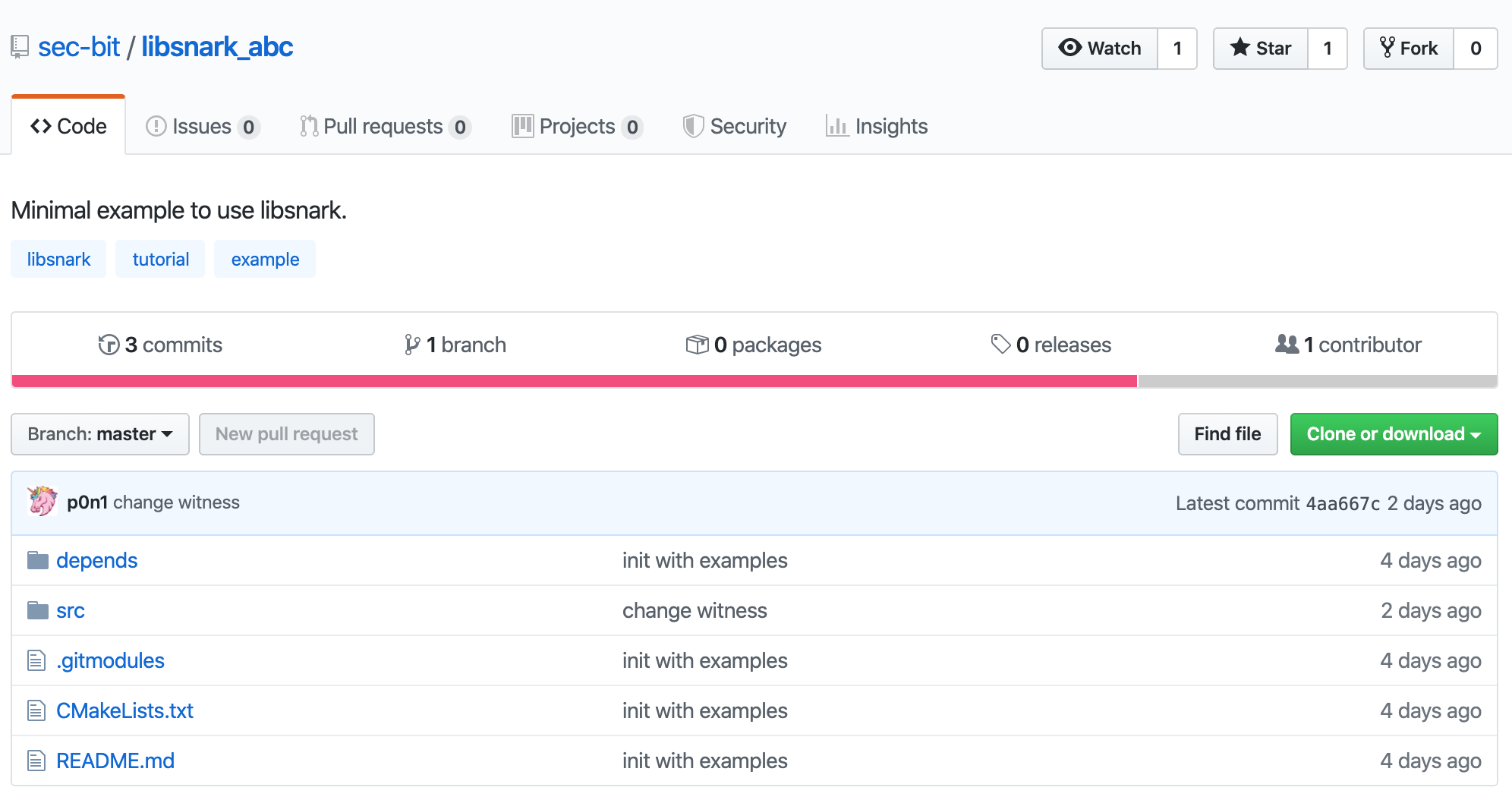1 2  cd libsnark_abc git submodule update --init --recursive 

 1  sudo apt-get install build-essential cmake git libgmp3-dev libprocps4-dev python-markdown libboost-all-dev libssl-dev 

 1  mkdir build && cd build && cmake .. 

 1  mkdir build && cd build && CPPFLAGS=-I/usr/local/opt/openssl/include LDFLAGS=-L/usr/local/opt/openssl/lib PKG_CONFIG_PATH=/usr/local/opt/openssl/lib/pkgconfig cmake -DWITH_PROCPS=OFF -DWITH_SUPERCOP=OFF .. 

 1  make 

 1 2 3  main range test 

 1  ./src/main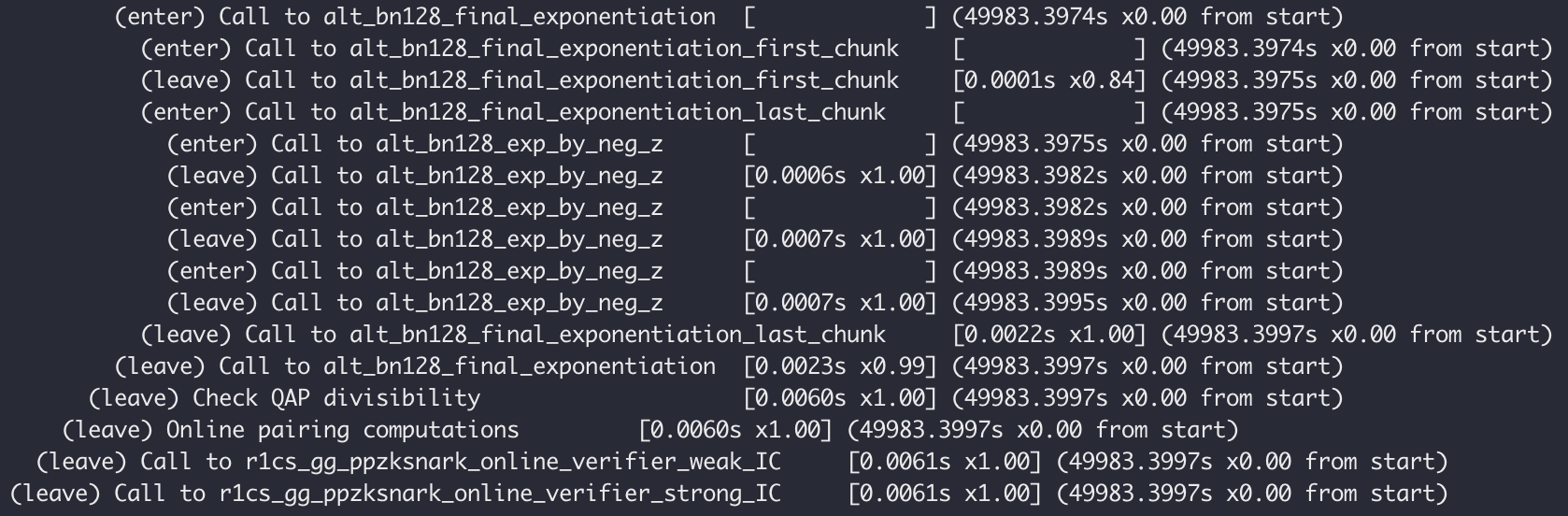## 4. 理解示例代码

 1 2 3 4 5 6 7 8 9  r1cs_gg_ppzksnark_keypair keypair = r1cs_gg_ppzksnark_generator(example.constraint_system); r1cs_gg_ppzksnark_processed_verification_key pvk = r1cs_gg_ppzksnark_verifier_process_vk(keypair.vk); r1cs_gg_ppzksnark_proof proof = r1cs_gg_ppzksnark_prover(keypair.pk, example.primary_input, example.auxiliary_input); const bool ans = r1cs_gg_ppzksnark_verifier_strong_IC(keypair.vk, example.primary_input, proof); const bool ans2 = r1cs_gg_ppzksnark_online_verifier_strong_IC(pvk, example.primary_input, proof); 

 1 2 3  #include #include #include 

 1 2  typedef libff::Fr FieldT; default_r1cs_gg_ppzksnark_pp::init_public_params(); 

 1 2 3 4  x * x = sym_1 sym_1 * x = y y + x = sym_2 sym_2 + 5 = out 

 1 2 3 4 5 6 7 8 9  // Create protoboard protoboard pb; // Define variables pb_variable x; pb_variable sym_1; pb_variable y; pb_variable sym_2; pb_variable out; 

 1 2 3 4 5 6  out.allocate(pb, "out"); x.allocate(pb, "x"); sym_1.allocate(pb, "sym_1"); y.allocate(pb, "y"); sym_2.allocate(pb, "sym_2"); pb.set_input_sizes(1); 

 1 2 3 4 5 6 7 8  // x*x = sym_1 pb.add_r1cs_constraint(r1cs_constraint(x, x, sym_1)); // sym_1 * x = y pb.add_r1cs_constraint(r1cs_constraint(sym_1, x, y)); // y + x = sym_2 pb.add_r1cs_constraint(r1cs_constraint(y + x, 1, sym_2)); // sym_2 + 5 = ~out pb.add_r1cs_constraint(r1cs_constraint(sym_2 + 5, 1, out)); 

 1 2  const r1cs_constraint_system constraint_system = pb.get_constraint_system(); const r1cs_gg_ppzksnark_keypair keypair = r1cs_gg_ppzksnark_generator(constraint_system); 

 1 2 3 4 5 6  pb.val(out) = 35; pb.val(x) = 3; pb.val(sym_1) = 9; pb.val(y) = 27; pb.val(sym_2) = 30; 

 1  const r1cs_gg_ppzksnark_proof proof = r1cs_gg_ppzksnark_prover(keypair.pk, pb.primary_input(), pb.auxiliary_input()); 

 1  bool verified = r1cs_gg_ppzksnark_verifier_strong_IC(keypair.vk, pb.primary_input(), proof);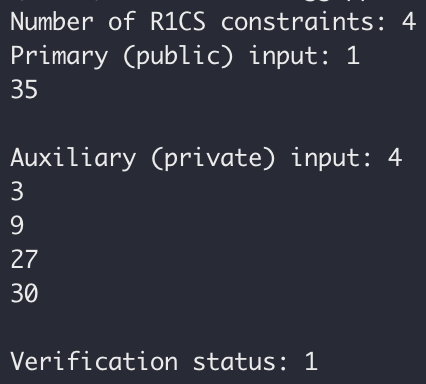## 5. 再次上手实践

zk-SNARKs 电路开发的主要工作量和难点在于如何用代码“精确”地描述命题中的所有约束。一旦描述不“精确”，则要么是漏掉约束、要么是写错约束，最终电路想要证明的内容则会与原命题相差甚远。上一节的例子只涉及简单的乘法和加法，与 r1cs_constraint 最基本的形式一致，因此约束的表达相对容易。除此之外几乎所有的约束都不是很直观，作为初学者很难正确地描述约束细节。

DangDangDanggadgetlib1/gadgets/basic_gadgets.hpp 中的 comparison_gadget 正是我们所需。

 1 2 3 4 5 6 7  comparison_gadget(protoboard& pb, const size_t n, const pb_linear_combination &A, const pb_linear_combination &B, const pb_variable &less, const pb_variable &less_or_eq, const std::string &annotation_prefix="") 

 1 2 3 4 5 6 7 8 9  protoboard pb; pb_variable x, max; pb_variable less, less_or_eq; x.allocate(pb, "x"); max.allocate(pb, "max"); pb.val(max)= 60; 

 1 2 3  comparison_gadget cmp(pb, 10, x, max, less, less_or_eq, "cmp"); cmp.generate_r1cs_constraints(); pb.add_r1cs_constraint(r1cs_constraint(less, 1, FieldT::one())); 

 1 2 3  // Add witness values pb.val(x) = 18; // secret cmp.generate_r1cs_witness(); 

## 7. 附录

### main.cpp

  1 2 3 4 5 6 7 8 9 10 11 12 13 14 15 16 17 18 19 20 21 22 23 24 25 26 27 28 29 30 31 32 33 34 35 36 37 38 39 40 41 42 43 44 45 46 47 48 49 50 51 52 53 54 55 56 57 58 59  #include #include #include #include using namespace libsnark; /** * The code below provides an example of all stages of running a R1CS GG-ppzkSNARK. * * Of course, in a real-life scenario, we would have three distinct entities, * mangled into one in the demonstration below. The three entities are as follows. * (1) The "generator", which runs the ppzkSNARK generator on input a given * constraint system CS to create a proving and a verification key for CS. * (2) The "prover", which runs the ppzkSNARK prover on input the proving key, * a primary input for CS, and an auxiliary input for CS. * (3) The "verifier", which runs the ppzkSNARK verifier on input the verification key, * a primary input for CS, and a proof. */ template bool run_r1cs_gg_ppzksnark(const r1cs_example > &example) { libff::print_header("R1CS GG-ppzkSNARK Generator"); r1cs_gg_ppzksnark_keypair keypair = r1cs_gg_ppzksnark_generator(example.constraint_system); printf("\n"); libff::print_indent(); libff::print_mem("after generator"); libff::print_header("Preprocess verification key"); r1cs_gg_ppzksnark_processed_verification_key pvk = r1cs_gg_ppzksnark_verifier_process_vk(keypair.vk); libff::print_header("R1CS GG-ppzkSNARK Prover"); r1cs_gg_ppzksnark_proof proof = r1cs_gg_ppzksnark_prover(keypair.pk, example.primary_input, example.auxiliary_input); printf("\n"); libff::print_indent(); libff::print_mem("after prover"); libff::print_header("R1CS GG-ppzkSNARK Verifier"); const bool ans = r1cs_gg_ppzksnark_verifier_strong_IC(keypair.vk, example.primary_input, proof); printf("\n"); libff::print_indent(); libff::print_mem("after verifier"); printf("* The verification result is: %s\n", (ans ? "PASS" : "FAIL")); libff::print_header("R1CS GG-ppzkSNARK Online Verifier"); const bool ans2 = r1cs_gg_ppzksnark_online_verifier_strong_IC(pvk, example.primary_input, proof); assert(ans == ans2); return ans; } template void test_r1cs_gg_ppzksnark(size_t num_constraints, size_t input_size) { r1cs_example > example = generate_r1cs_example_with_binary_input >(num_constraints, input_size); const bool bit = run_r1cs_gg_ppzksnark(example); assert(bit); } int main () { default_r1cs_gg_ppzksnark_pp::init_public_params(); test_r1cs_gg_ppzksnark(1000, 100); return 0; } 

### test.cpp

  1 2 3 4 5 6 7 8 9 10 11 12 13 14 15 16 17 18 19 20 21 22 23 24 25 26 27 28 29 30 31 32 33 34 35 36 37 38 39 40 41 42 43 44 45 46 47 48 49 50 51 52 53 54 55 56 57 58 59 60 61 62 63 64 65 66 67 68 69 70 71 72 73 74  #include #include #include using namespace libsnark; using namespace std; int main () { typedef libff::Fr FieldT; // Initialize the curve parameters default_r1cs_gg_ppzksnark_pp::init_public_params(); // Create protoboard protoboard pb; // Define variables pb_variable x; pb_variable sym_1; pb_variable y; pb_variable sym_2; pb_variable out; // Allocate variables to protoboard // The strings (like "x") are only for debugging purposes out.allocate(pb, "out"); x.allocate(pb, "x"); sym_1.allocate(pb, "sym_1"); y.allocate(pb, "y"); sym_2.allocate(pb, "sym_2"); // This sets up the protoboard variables // so that the first one (out) represents the public // input and the rest is private input pb.set_input_sizes(1); // Add R1CS constraints to protoboard // x*x = sym_1 pb.add_r1cs_constraint(r1cs_constraint(x, x, sym_1)); // sym_1 * x = y pb.add_r1cs_constraint(r1cs_constraint(sym_1, x, y)); // y + x = sym_2 pb.add_r1cs_constraint(r1cs_constraint(y + x, 1, sym_2)); // sym_2 + 5 = ~out pb.add_r1cs_constraint(r1cs_constraint(sym_2 + 5, 1, out)); const r1cs_constraint_system constraint_system = pb.get_constraint_system(); // generate keypair const r1cs_gg_ppzksnark_keypair keypair = r1cs_gg_ppzksnark_generator(constraint_system); // Add public input and witness values pb.val(out) = 35; pb.val(x) = 3; pb.val(sym_1) = 9; pb.val(y) = 27; pb.val(sym_2) = 30; // generate proof const r1cs_gg_ppzksnark_proof proof = r1cs_gg_ppzksnark_prover(keypair.pk, pb.primary_input(), pb.auxiliary_input()); // verify bool verified = r1cs_gg_ppzksnark_verifier_strong_IC(keypair.vk, pb.primary_input(), proof); cout << "Number of R1CS constraints: " << constraint_system.num_constraints() << endl; cout << "Primary (public) input: " << pb.primary_input() << endl; cout << "Auxiliary (private) input: " << pb.auxiliary_input() << endl; cout << "Verification status: " << verified << endl; } 

### range.cpp

  1 2 3 4 5 6 7 8 9 10 11 12 13 14 15 16 17 18 19 20 21 22 23 24 25 26 27 28 29 30 31 32 33 34 35 36 37 38 39 40 41 42 43 44 45 46 47 48 49  #include #include #include #include using namespace libsnark; using namespace std; int main () { typedef libff::Fr FieldT; // Initialize the curve parameters default_r1cs_gg_ppzksnark_pp::init_public_params(); // Create protoboard protoboard pb; pb_variable x, max; pb_variable less, less_or_eq; x.allocate(pb, "x"); max.allocate(pb, "max"); pb.val(max)= 60; comparison_gadget cmp(pb, 10, x, max, less, less_or_eq, "cmp"); cmp.generate_r1cs_constraints(); pb.add_r1cs_constraint(r1cs_constraint(less, 1, FieldT::one())); const r1cs_constraint_system constraint_system = pb.get_constraint_system(); // generate keypair const r1cs_gg_ppzksnark_keypair keypair = r1cs_gg_ppzksnark_generator(constraint_system); // Add witness values pb.val(x) = 18; // secret cmp.generate_r1cs_witness(); // generate proof const r1cs_gg_ppzksnark_proof proof = r1cs_gg_ppzksnark_prover(keypair.pk, pb.primary_input(), pb.auxiliary_input()); // verify bool verified = r1cs_gg_ppzksnark_verifier_strong_IC(keypair.vk, pb.primary_input(), proof); cout << "Number of R1CS constraints: " << constraint_system.num_constraints() << endl; cout << "Primary (public) input: " << pb.primary_input() << endl; cout << "Auxiliary (private) input: " << pb.auxiliary_input() << endl; cout << "Verification status: " << verified << endl; }